Successfully reported this slideshow.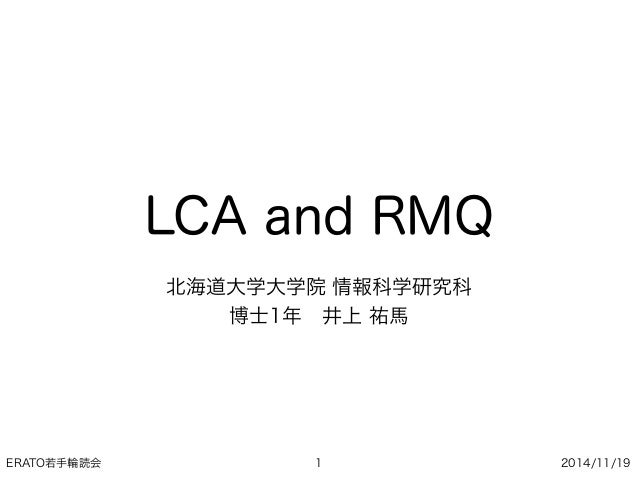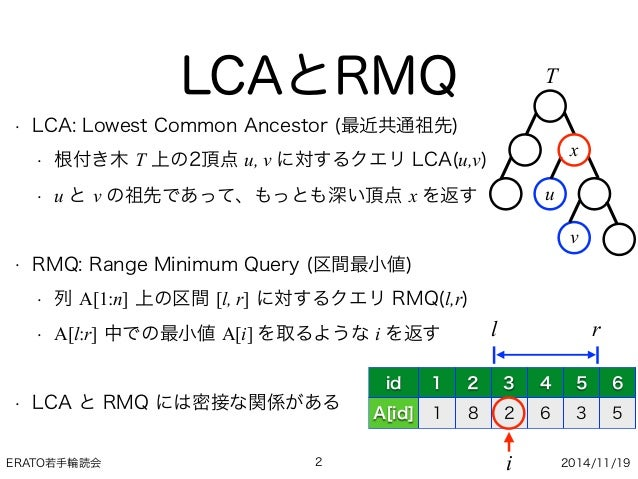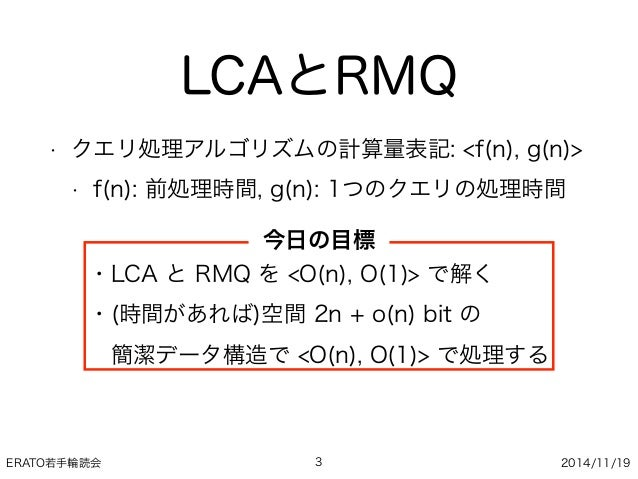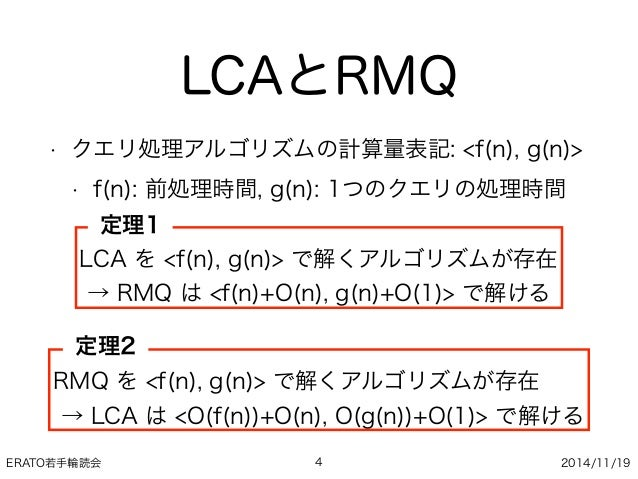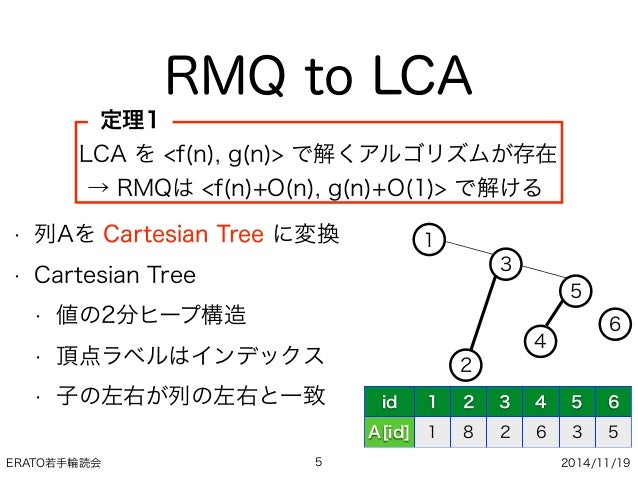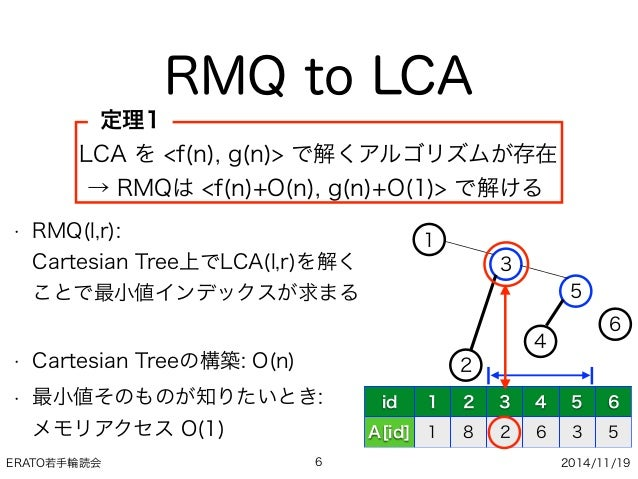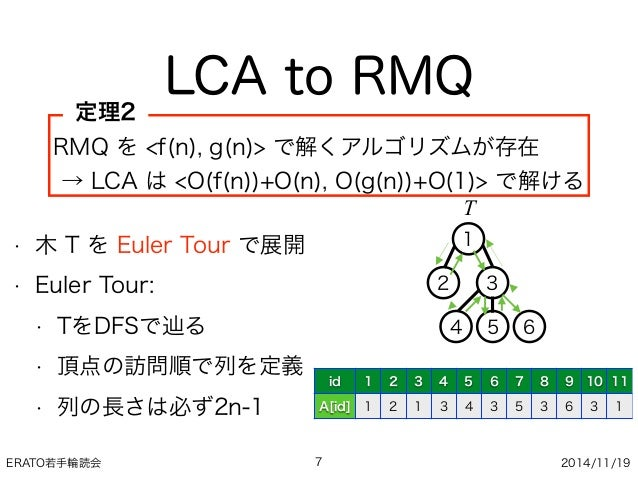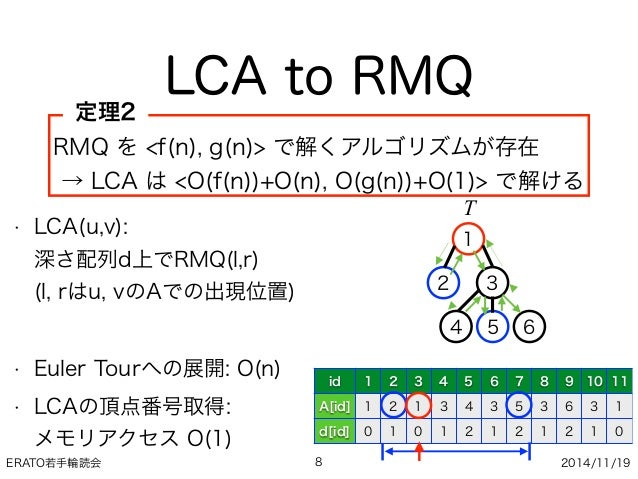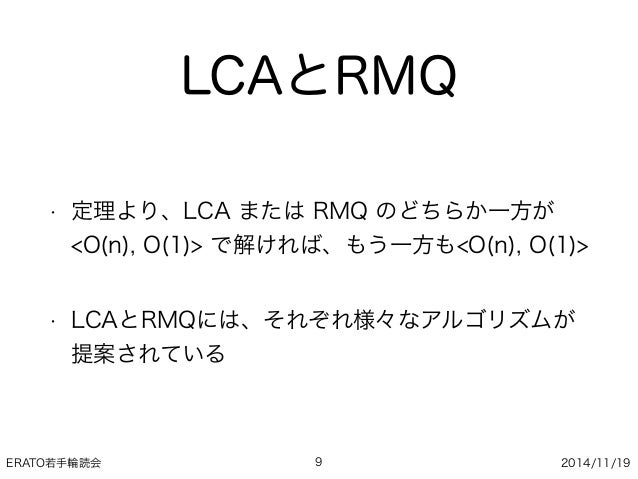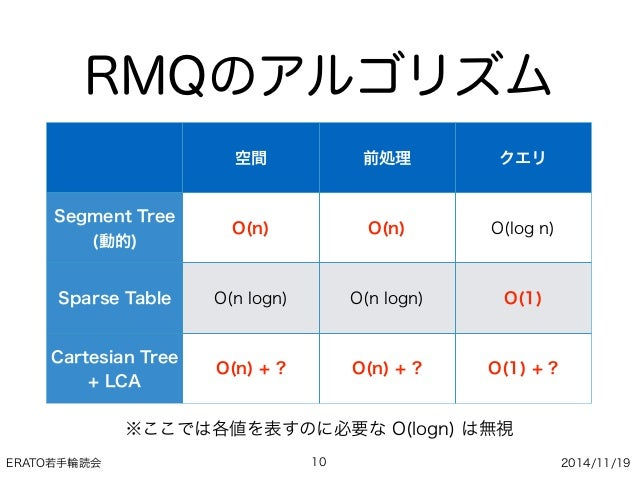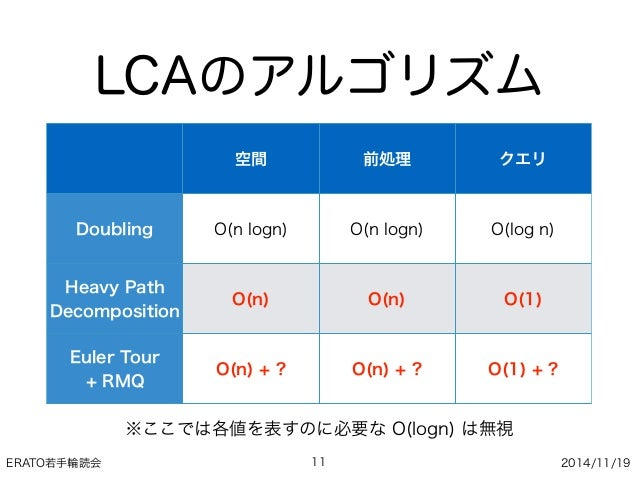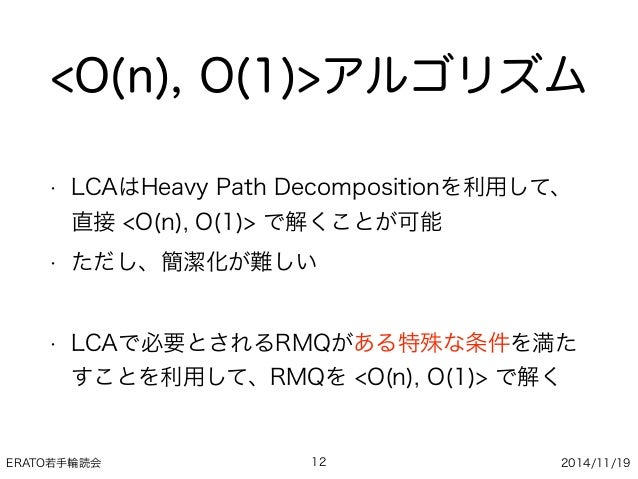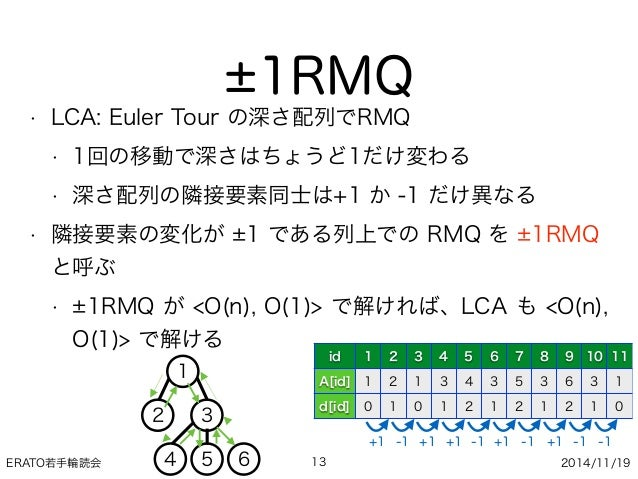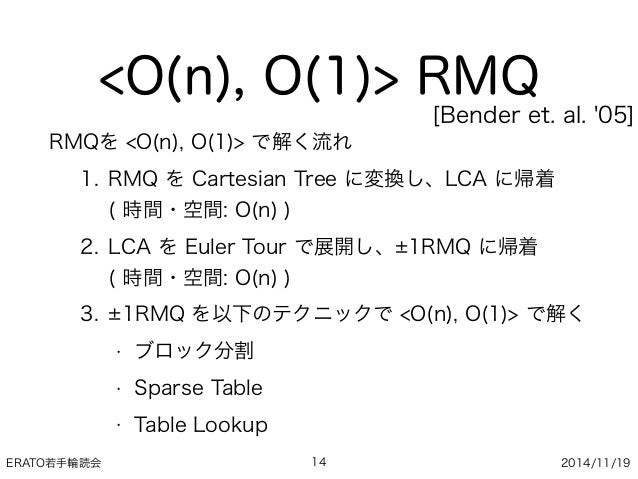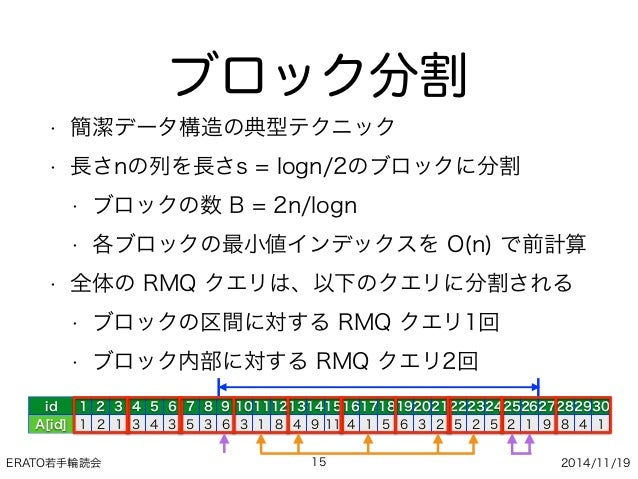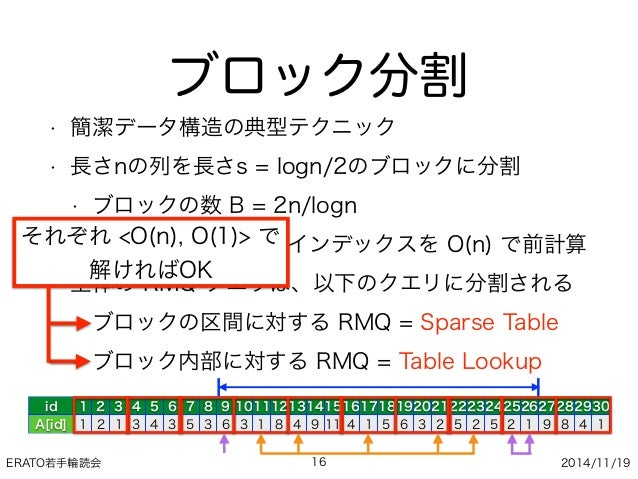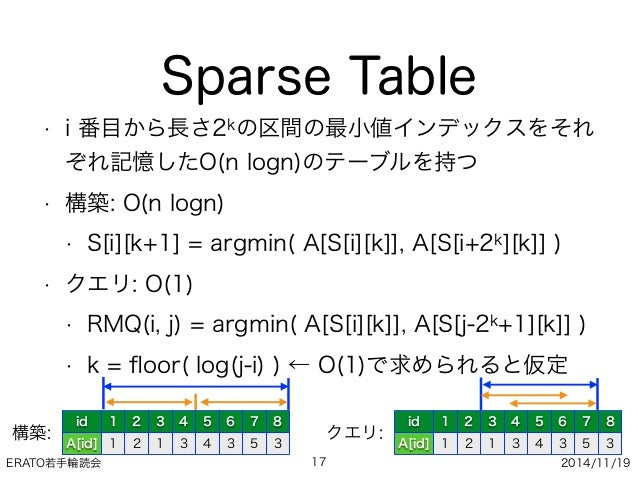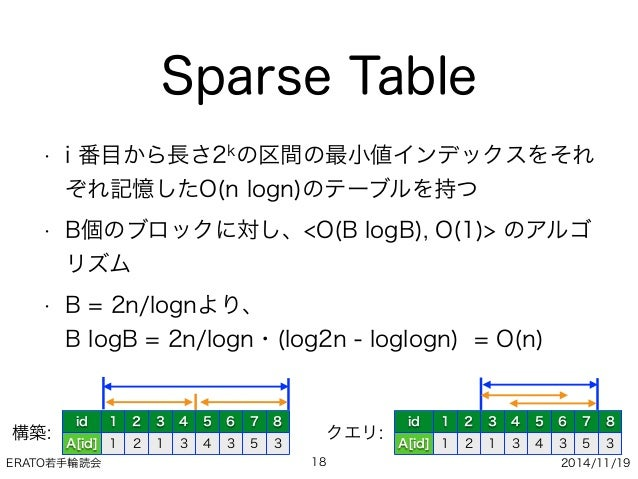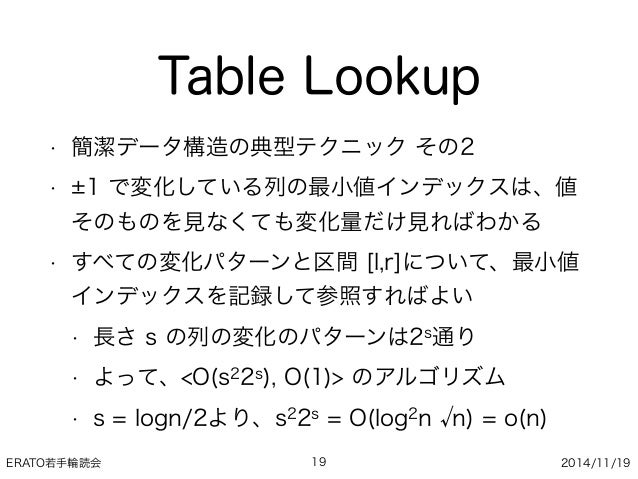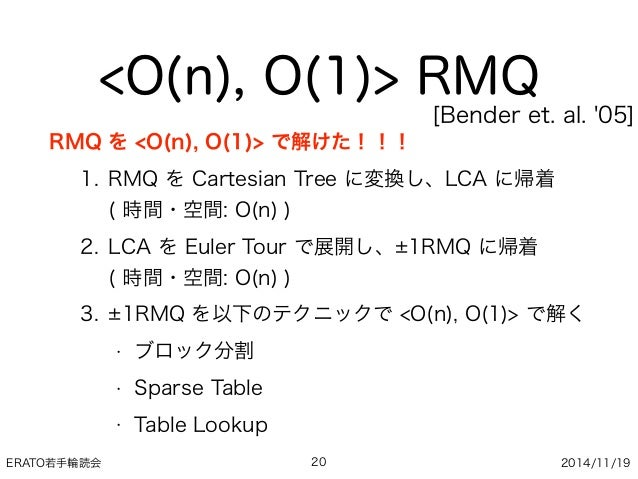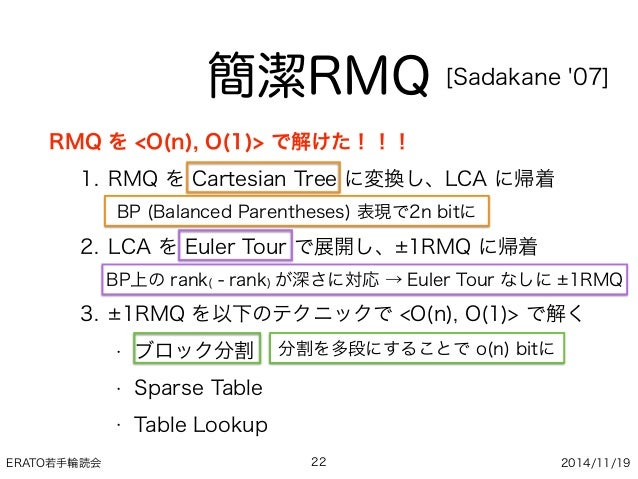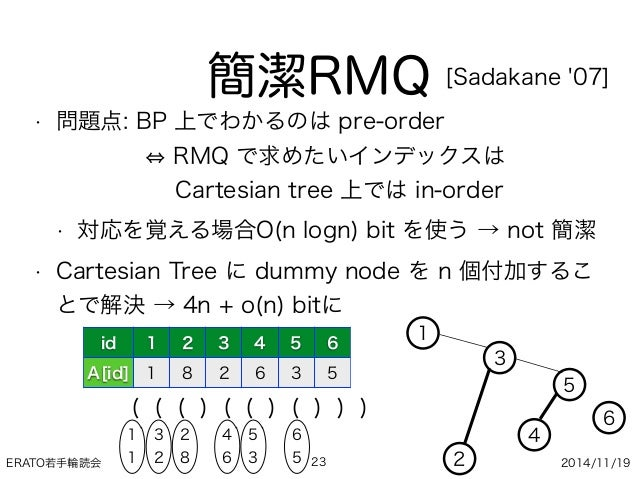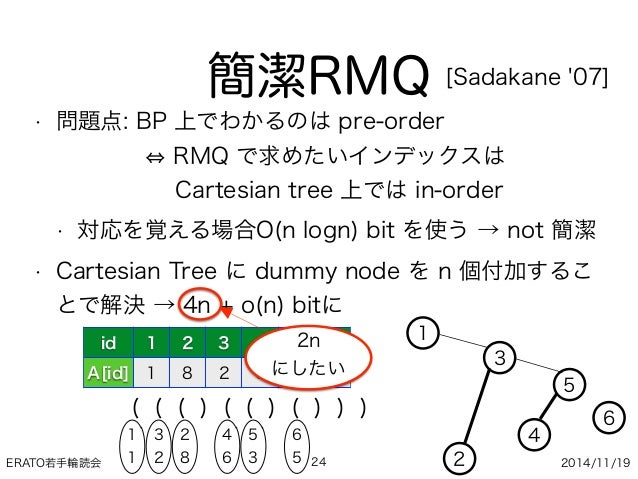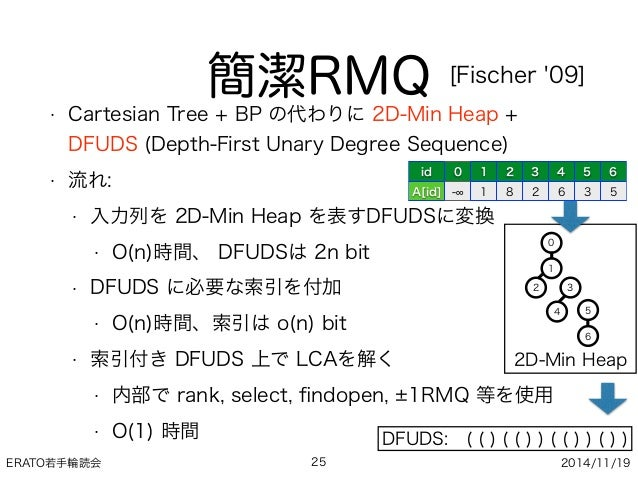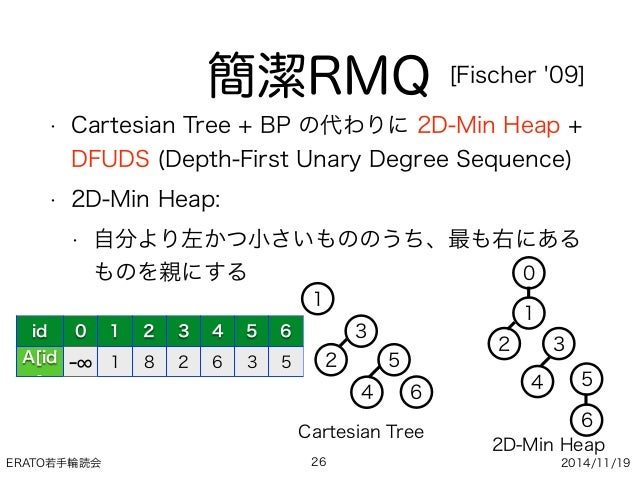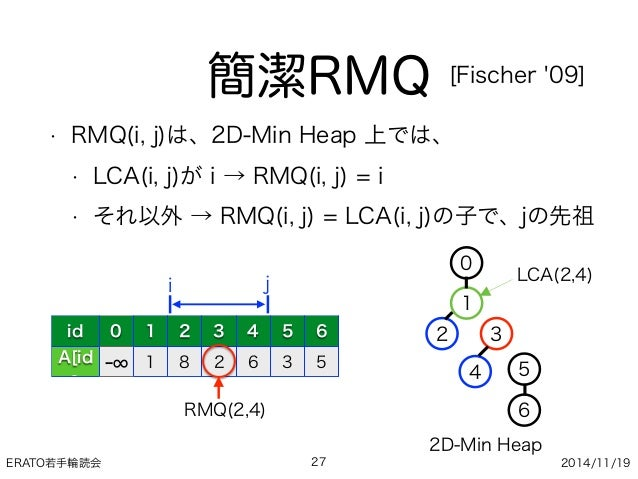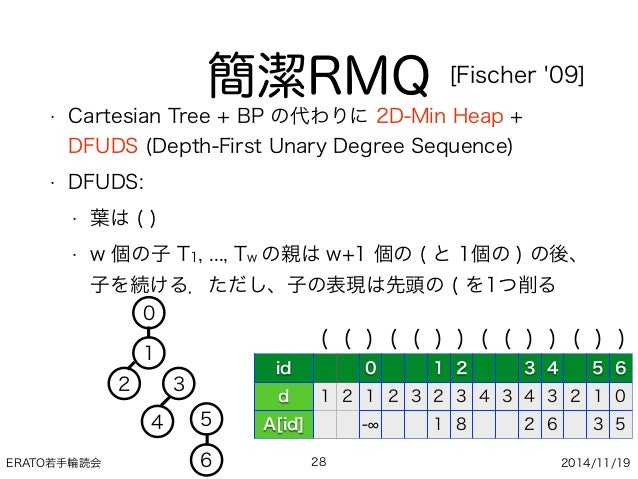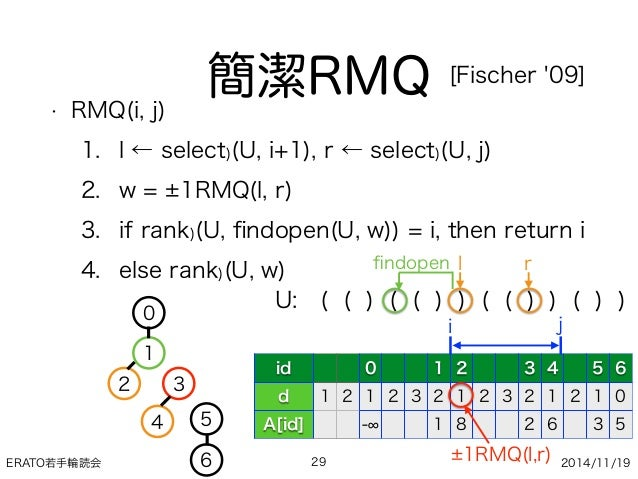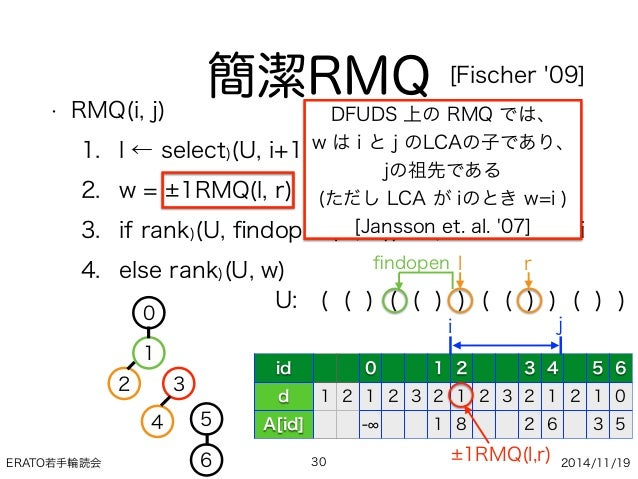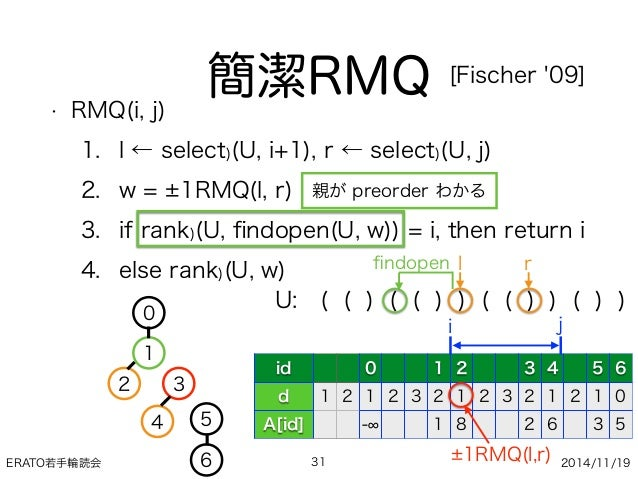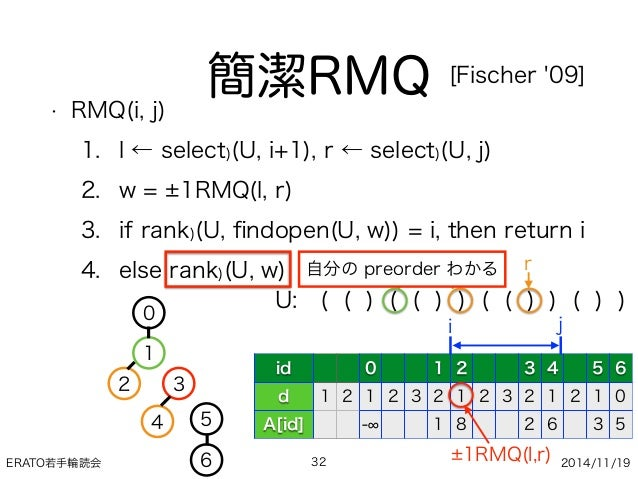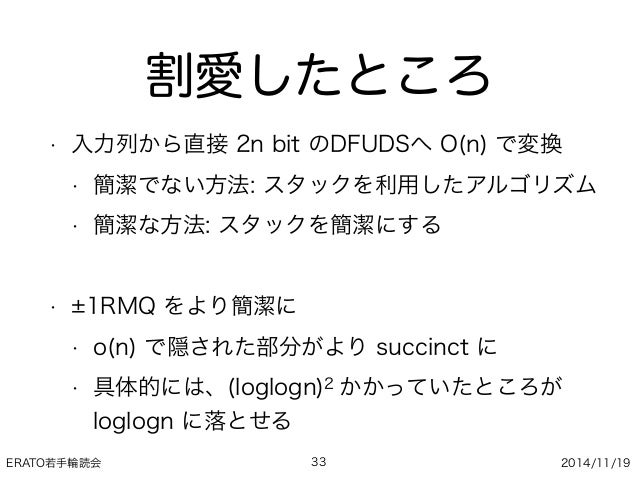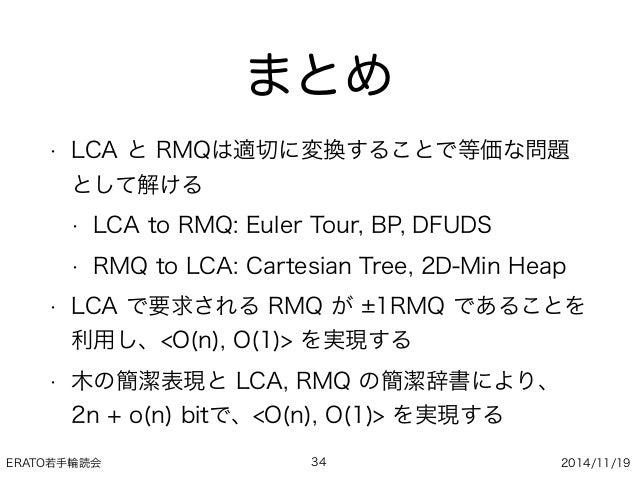Upcoming SlideShare
×

# LCA and RMQ ~簡潔もあるよ！~

LCAとRMQの関係とそれを利用した高速なアルゴリズム、簡潔データ構造化の大雑把な解説。

• Full Name
Comment goes here.

Are you sure you want to Yes No• Be the first to comment

### LCA and RMQ ~簡潔もあるよ！~

1. 1. ERATO若手輪読会 2014/11/19 LCA and RMQ 北海道大学大学院 情報科学研究科 博士1年 井上 祐馬 1
2. 2. ERATO若手輪読会 2014/11/19 • LCA: Lowest Common Ancestor (最近共通祖先) • 根付き木 T 上の2頂点 u, v に対するクエリ LCA(u,v) • u と v の祖先であって、もっとも深い頂点 x を返す • RMQ: Range Minimum Query (区間最小値) • 列 A[1:n] 上の区間 [l, r] に対するクエリ RMQ(l,r) • A[l:r] 中での最小値 A[i] を取るような i を返す • LCA と RMQ には密接な関係がある LCAとRMQ 2 u v x id 1 2 3 4 5 6 A[id] 1 8 2 6 3 5 l r i T
3. 3. ERATO若手輪読会 2014/11/19 LCAとRMQ • クエリ処理アルゴリズムの計算量表記: <f(n), g(n)> • f(n): 前処理時間, g(n): 1つのクエリの処理時間 3 ・LCA と RMQ を <O(n), O(1)> で解く ・(時間があれば)空間 2n + o(n) bit の  簡潔データ構造で <O(n), O(1)> で処理する 今日の目標
4. 4. ERATO若手輪読会 2014/11/19 LCAとRMQ • クエリ処理アルゴリズムの計算量表記: <f(n), g(n)> • f(n): 前処理時間, g(n): 1つのクエリの処理時間 4 LCA を <f(n), g(n)> で解くアルゴリズムが存在  → RMQ は <f(n)+O(n), g(n)+O(1)> で解ける RMQ を <f(n), g(n)> で解くアルゴリズムが存在  → LCA は <O(f(n))+O(n), O(g(n))+O(1)> で解ける 定理1 定理2
5. 5. ERATO若手輪読会 2014/11/19 RMQ to LCA 5 LCA を <f(n), g(n)> で解くアルゴリズムが存在  → RMQは <f(n)+O(n), g(n)+O(1)> で解ける 定理1 • 列Aを Cartesian Tree に変換 • Cartesian Tree • 値の2分ヒープ構造 • 頂点ラベルはインデックス • 子の左右が列の左右と一致 id 1 2 3 4 5 6 A[id] 1 8 2 6 3 5 1 3 2 5 4 6
6. 6. ERATO若手輪読会 2014/11/19 RMQ to LCA 6 • RMQ(l,r):  Cartesian Tree上でLCA(l,r)を解く ことで最小値インデックスが求まる  • Cartesian Treeの構築: O(n) • 最小値そのものが知りたいとき:  メモリアクセス O(1) id 1 2 3 4 5 6 A[id] 1 8 2 6 3 5 1 3 2 5 4 6 LCA を <f(n), g(n)> で解くアルゴリズムが存在  → RMQは <f(n)+O(n), g(n)+O(1)> で解ける 定理1
7. 7. ERATO若手輪読会 2014/11/19 LCA to RMQ • 木 T を Euler Tour で展開 • Euler Tour: • TをDFSで辿る • 頂点の訪問順で列を定義 • 列の長さは必ず2n-1 7 RMQ を <f(n), g(n)> で解くアルゴリズムが存在  → LCA は <O(f(n))+O(n), O(g(n))+O(1)> で解ける 定理2 4 65 2 1 3 T id 1 2 3 4 5 6 7 8 9 10 11 A[id] 1 2 1 3 4 3 5 3 6 3 1
8. 8. ERATO若手輪読会 2014/11/19 LCA to RMQ • LCA(u,v):  深さ配列d上でRMQ(l,r)  (l, rはu, vのAでの出現位置) • Euler Tourへの展開: O(n) • LCAの頂点番号取得:   メモリアクセス O(1) 8 RMQ を <f(n), g(n)> で解くアルゴリズムが存在  → LCA は <O(f(n))+O(n), O(g(n))+O(1)> で解ける 定理2 4 65 2 1 3 T id 1 2 3 4 5 6 7 8 9 10 11 A[id] 1 2 1 3 4 3 5 3 6 3 1 d[id] 0 1 0 1 2 1 2 1 2 1 0
9. 9. ERATO若手輪読会 2014/11/19 LCAとRMQ • 定理より、LCA または RMQ のどちらか一方が <O(n), O(1)> で解ければ、もう一方も<O(n), O(1)> • LCAとRMQには、それぞれ様々なアルゴリズムが 提案されている 9
10. 10. ERATO若手輪読会 2014/11/19 RMQのアルゴリズム 10 空間 前処理 クエリ Segment Tree (動的) O(n) O(n) O(log n) Sparse Table O(n logn) O(n logn) O(1) Cartesian Tree + LCA O(n) + ? O(n) + ? O(1) + ? ※ここでは各値を表すのに必要な O(logn) は無視
11. 11. ERATO若手輪読会 2014/11/19 LCAのアルゴリズム 11 空間 前処理 クエリ Doubling O(n logn) O(n logn) O(log n) Heavy Path Decomposition O(n) O(n) O(1) Euler Tour + RMQ O(n) + ? O(n) + ? O(1) + ? ※ここでは各値を表すのに必要な O(logn) は無視
12. 12. ERATO若手輪読会 2014/11/19 <O(n), O(1)>アルゴリズム • LCAはHeavy Path Decompositionを利用して、 直接 <O(n), O(1)> で解くことが可能 • ただし、簡潔化が難しい • LCAで必要とされるRMQがある特殊な条件を満た すことを利用して、RMQを <O(n), O(1)> で解く 12
13. 13. ERATO若手輪読会 2014/11/19 1RMQ • LCA: Euler Tour の深さ配列でRMQ • 1回の移動で深さはちょうど1だけ変わる • 深さ配列の隣接要素同士は+1 か -1 だけ異なる • 隣接要素の変化が 1 である列上での RMQ を 1RMQ と呼ぶ • 1RMQ が <O(n), O(1)> で解ければ、LCA も <O(n), O(1)> で解ける 134 65 2 1 3 id 1 2 3 4 5 6 7 8 9 10 11 A[id] 1 2 1 3 4 3 5 3 6 3 1 d[id] 0 1 0 1 2 1 2 1 2 1 0 +1 +1 +1 +1 +1 -1 -1-1-1-1
14. 14. ERATO若手輪読会 2014/11/19 <O(n), O(1)> RMQ RMQを <O(n), O(1)> で解く流れ 1. RMQ を Cartesian Tree に変換し、LCA に帰着  ( 時間・空間: O(n) ) 2. LCA を Euler Tour で展開し、 1RMQ に帰着  ( 時間・空間: O(n) ) 3. 1RMQ を以下のテクニックで <O(n), O(1)> で解く • ブロック分割 • Sparse Table • Table Lookup 14 [Bender et. al. '05]
15. 15. ERATO若手輪読会 2014/11/19 ブロック分割 • 簡潔データ構造の典型テクニック • 長さnの列を長さs = logn/2のブロックに分割 • ブロックの数 B = 2n/logn • 各ブロックの最小値インデックスを O(n) で前計算 • 全体の RMQ クエリは、以下のクエリに分割される • ブロックの区間に対する RMQ クエリ1回 • ブロック内部に対する RMQ クエリ2回 15 id 1 2 3 4 5 6 7 8 9 101112131415161718192021222324252627282930 A[id] 1 2 1 3 4 3 5 3 6 3 1 8 4 9 11 4 1 5 6 3 2 5 2 5 2 1 9 8 4 1
16. 16. ERATO若手輪読会 2014/11/19 ブロック分割 • 簡潔データ構造の典型テクニック • 長さnの列を長さs = logn/2のブロックに分割 • ブロックの数 B = 2n/logn • 各ブロックの最小値インデックスを O(n) で前計算 • 全体の RMQ クエリは、以下のクエリに分割される • ブロックの区間に対する RMQ = Sparse Table • ブロック内部に対する RMQ = Table Lookup 16 id 1 2 3 4 5 6 7 8 9 101112131415161718192021222324252627282930 A[id] 1 2 1 3 4 3 5 3 6 3 1 8 4 9 11 4 1 5 6 3 2 5 2 5 2 1 9 8 4 1 それぞれ <O(n), O(1)> で 解ければOK
17. 17. ERATO若手輪読会 2014/11/19 Sparse Table • i 番目から長さ2kの区間の最小値インデックスをそれ ぞれ記憶したO(n logn)のテーブルを持つ • 構築: O(n logn) • S[i][k+1] = argmin( A[S[i][k]], A[S[i+2k][k]] ) • クエリ: O(1) • RMQ(i, j) = argmin( A[S[i][k]], A[S[j-2k+1][k]] ) • k = ﬂoor( log(j-i) ) ← O(1)で求められると仮定 17 id 1 2 3 4 5 6 7 8 A[id] 1 2 1 3 4 3 5 3 id 1 2 3 4 5 6 7 8 A[id] 1 2 1 3 4 3 5 3 構築: クエリ:
18. 18. ERATO若手輪読会 2014/11/19 Sparse Table • i 番目から長さ2kの区間の最小値インデックスをそれ ぞれ記憶したO(n logn)のテーブルを持つ • B個のブロックに対し、<O(B logB), O(1)> のアルゴ リズム • B = 2n/lognより、  B logB = 2n/logn・(log2n - loglogn) = O(n) 18 id 1 2 3 4 5 6 7 8 A[id] 1 2 1 3 4 3 5 3 id 1 2 3 4 5 6 7 8 A[id] 1 2 1 3 4 3 5 3 構築: クエリ:
19. 19. ERATO若手輪読会 2014/11/19 Table Lookup • 簡潔データ構造の典型テクニック その2 • 1 で変化している列の最小値インデックスは、値 そのものを見なくても変化量だけ見ればわかる • すべての変化パターンと区間 [l,r]について、最小値 インデックスを記録して参照すればよい • 長さ s の列の変化のパターンは2s通り • よって、<O(s22s), O(1)> のアルゴリズム • s = logn/2より、s22s = O(log2n n) = o(n) 19
20. 20. ERATO若手輪読会 2014/11/19 <O(n), O(1)> RMQ RMQ を <O(n), O(1)> で解けた！！！ 1. RMQ を Cartesian Tree に変換し、LCA に帰着  ( 時間・空間: O(n) ) 2. LCA を Euler Tour で展開し、 1RMQ に帰着  ( 時間・空間: O(n) ) 3. 1RMQ を以下のテクニックで <O(n), O(1)> で解く • ブロック分割 • Sparse Table • Table Lookup 20 [Bender et. al. '05]
21. 21. ERATO若手輪読会 2014/11/19 -----↓ここから簡潔↓----- 21
22. 22. ERATO若手輪読会 2014/11/19 簡潔RMQ RMQ を <O(n), O(1)> で解けた！！！ 1. RMQ を Cartesian Tree に変換し、LCA に帰着  ( 時間・空間: O(n) ) 2. LCA を Euler Tour で展開し、 1RMQ に帰着  ( 時間・空間: O(n) ) 3. 1RMQ を以下のテクニックで <O(n), O(1)> で解く • ブロック分割 • Sparse Table • Table Lookup 22 BP (Balanced Parentheses) 表現で2n bitに BP上の rank( - rank) が深さに対応 → Euler Tour なしに 1RMQ 分割を多段にすることで o(n) bitに [Sadakane '07]
23. 23. ERATO若手輪読会 2014/11/19 簡潔RMQ • 問題点: BP 上でわかるのは pre-order  RMQ で求めたいインデックスは  Cartesian tree 上では in-order • 対応を覚える場合O(n logn) bit を使う → not 簡潔 • Cartesian Tree に dummy node を n 個付加するこ とで解決 → 4n + o(n) bitに 23 [Sadakane '07] id 1 2 3 4 5 6 A[id] 1 8 2 6 3 5 1 3 2 5 4 6 ( ( ( ) ( ( ) ( ) ) ) 1 1 3 2 2 8 4 6 5 3 6 5
24. 24. ERATO若手輪読会 2014/11/19 簡潔RMQ • 問題点: BP 上でわかるのは pre-order  RMQ で求めたいインデックスは  Cartesian tree 上では in-order • 対応を覚える場合O(n logn) bit を使う → not 簡潔 • Cartesian Tree に dummy node を n 個付加するこ とで解決 → 4n + o(n) bitに 24 [Sadakane '07] id 1 2 3 4 5 6 A[id] 1 8 2 6 3 5 1 3 2 5 4 6 ( ( ( ) ( ( ) ( ) ) ) 1 1 3 2 2 8 4 6 5 3 6 5 2n にしたい
25. 25. ERATO若手輪読会 2014/11/19 簡潔RMQ • Cartesian Tree + BP の代わりに 2D-Min Heap + DFUDS (Depth-First Unary Degree Sequence) • 流れ: • 入力列を 2D-Min Heap を表すDFUDSに変換 • O(n)時間、 DFUDSは 2n bit • DFUDS に必要な索引を付加 • O(n)時間、索引は o(n) bit • 索引付き DFUDS 上で LCAを解く • 内部で rank, select, ﬁndopen, 1RMQ 等を使用 • O(1) 時間 25 [Fischer '09] id 0 1 2 3 4 5 6 A[id] - 1 8 2 6 3 5 1 32 54 6 2D-Min Heap 0 DFUDS: ( ( ) ( ( ) ) ( ( ) ) ( ) )
26. 26. ERATO若手輪読会 2014/11/19 簡潔RMQ • Cartesian Tree + BP の代わりに 2D-Min Heap + DFUDS (Depth-First Unary Degree Sequence) • 2D-Min Heap: • 自分より左かつ小さいもののうち、最も右にある ものを親にする 26 [Fischer '09] id 0 1 2 3 4 5 6 A[id ] - 1 8 2 6 3 5 1 3 2 5 4 6 1 32 54 6 Cartesian Tree 2D-Min Heap 0
27. 27. ERATO若手輪読会 2014/11/19 簡潔RMQ • RMQ(i, j)は、2D-Min Heap 上では、 • LCA(i, j)が i → RMQ(i, j) = i • それ以外 → RMQ(i, j) = LCA(i, j)の子で、jの先祖 27 [Fischer '09] id 0 1 2 3 4 5 6 A[id ] - 1 8 2 6 3 5 2D-Min Heap 1 32 54 6 0 i j LCA(2,4) RMQ(2,4)
28. 28. ERATO若手輪読会 2014/11/19 簡潔RMQ • Cartesian Tree + BP の代わりに 2D-Min Heap + DFUDS (Depth-First Unary Degree Sequence) • DFUDS: • 葉は ( ) • w 個の子 T1, ..., Tw の親は w+1 個の ( と 1個の ) の後、 子を続ける．ただし、子の表現は先頭の ( を1つ削る 28 [Fischer '09] ( ( ) ( ( ) ) ( ( ) ) ( ) ) id 0 1 2 3 4 5 6 d 1 2 1 2 3 2 3 4 3 4 3 2 1 0 A[id] - 1 8 2 6 3 5 1 32 54 6 0
29. 29. ERATO若手輪読会 2014/11/19 • RMQ(i, j) 1. l ← select)(U, i+1), r ← select)(U, j) 2. w = 1RMQ(l, r) 3. if rank)(U, ﬁndopen(U, w)) = i, then return i 4. else rank)(U, w) 29 簡潔RMQ [Fischer '09] U: ( ( ) ( ( ) ) ( ( ) ) ( ) ) id 0 1 2 3 4 5 6 d 1 2 1 2 3 2 1 2 3 2 1 2 1 0 A[id] - 1 8 2 6 3 5 1 32 54 6 0 i j l r 1RMQ(l,r) ﬁndopen
30. 30. ERATO若手輪読会 2014/11/19 • RMQ(i, j) 1. l ← select)(U, i+1), r ← select)(U, j) 2. w = 1RMQ(l, r) 3. if rank)(U, ﬁndopen(U, w)) = i, then return i 4. else rank)(U, w) 30 簡潔RMQ [Fischer '09] U: ( ( ) ( ( ) ) ( ( ) ) ( ) ) id 0 1 2 3 4 5 6 d 1 2 1 2 3 2 1 2 3 2 1 2 1 0 A[id] - 1 8 2 6 3 5 1 32 54 6 0 i j l r 1RMQ(l,r) ﬁndopen DFUDS 上の RMQ では、 w は i と j のLCAの子であり、 jの祖先である (ただし LCA が iのとき w=i ) [Jansson et. al. '07]
31. 31. ERATO若手輪読会 2014/11/19 • RMQ(i, j) 1. l ← select)(U, i+1), r ← select)(U, j) 2. w = 1RMQ(l, r) 3. if rank)(U, ﬁndopen(U, w)) = i, then return i 4. else rank)(U, w) 31 簡潔RMQ [Fischer '09] U: ( ( ) ( ( ) ) ( ( ) ) ( ) ) id 0 1 2 3 4 5 6 d 1 2 1 2 3 2 1 2 3 2 1 2 1 0 A[id] - 1 8 2 6 3 5 1 32 54 6 0 i j l r 1RMQ(l,r) ﬁndopen 親が preorder わかる
32. 32. ERATO若手輪読会 2014/11/19 • RMQ(i, j) 1. l ← select)(U, i+1), r ← select)(U, j) 2. w = 1RMQ(l, r) 3. if rank)(U, ﬁndopen(U, w)) = i, then return i 4. else rank)(U, w) 32 簡潔RMQ [Fischer '09] U: ( ( ) ( ( ) ) ( ( ) ) ( ) ) id 0 1 2 3 4 5 6 d 1 2 1 2 3 2 1 2 3 2 1 2 1 0 A[id] - 1 8 2 6 3 5 1 32 54 6 0 i j l r 1RMQ(l,r) ﬁndopen自分の preorder わかる
33. 33. ERATO若手輪読会 2014/11/19 割愛したところ • 入力列から直接 2n bit のDFUDSへ O(n) で変換 • 簡潔でない方法: スタックを利用したアルゴリズム • 簡潔な方法: スタックを簡潔にする • 1RMQ をより簡潔に • o(n) で隠された部分がより succinct に • 具体的には、(loglogn)2 かかっていたところが loglogn に落とせる 33
34. 34. ERATO若手輪読会 2014/11/19 まとめ • LCA と RMQは適切に変換することで等価な問題 として解ける • LCA to RMQ: Euler Tour, BP, DFUDS • RMQ to LCA: Cartesian Tree, 2D-Min Heap • LCA で要求される RMQ が 1RMQ であることを 利用し、<O(n), O(1)> を実現する • 木の簡潔表現と LCA, RMQ の簡潔辞書により、 2n + o(n) bitで、<O(n), O(1)> を実現する 34

### Be the first to comment

•#### ssuser9e1e6b

Apr. 7, 2015
•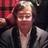#### KanSakamoto

Apr. 7, 2015
•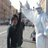#### keisukegoto92

Aug. 13, 2015
•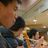#### TomoyaKita

Nov. 19, 2015
•#### ShinyaMiyagawa

Jan. 7, 2016
•#### ssuser0745d1

Oct. 20, 2016
•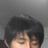#### toshimitsuuesaka

Nov. 2, 2016
•#### yasu89

Jan. 18, 2017
•#### ssuser31939b

Mar. 27, 2018
•#### JunpeiMishima

Jun. 4, 2018
•#### KernelMarker

Aug. 16, 2018
•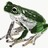#### KojiHigasa

Jun. 24, 2019
•#### tinsep19

Jul. 9, 2019
•Mar. 4, 2020

Total views

9,718

On Slideshare

0

From embeds

0

Number of embeds

731

39

Shares

0